#### 2-Wire LCD Board w/DS3234 RTC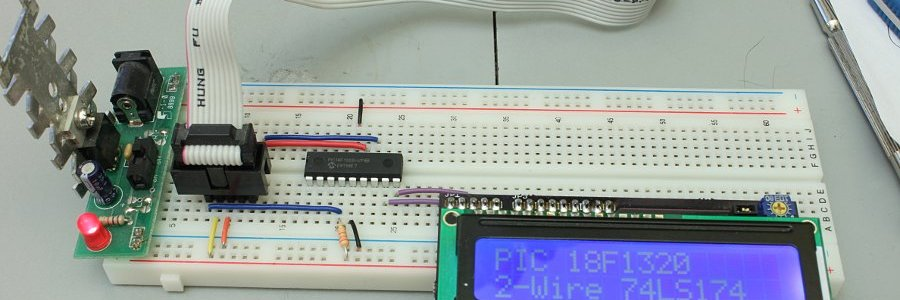I got tired of building the Myke Predko 2-Wire LCD circuit over and over and/or having one breadboard always used up so I'd have one handy. So I designed a PC board with the circuit on it. And, since I had tons of extra space under the LCD I added a Maxim DS3234 RTC and a battery holder under there.

Here are the boards (with some others that came in the same order), and a top view of the LCD board. The boards are, as usual, by DorkbotPDX, and are, as usual, of excellent quality.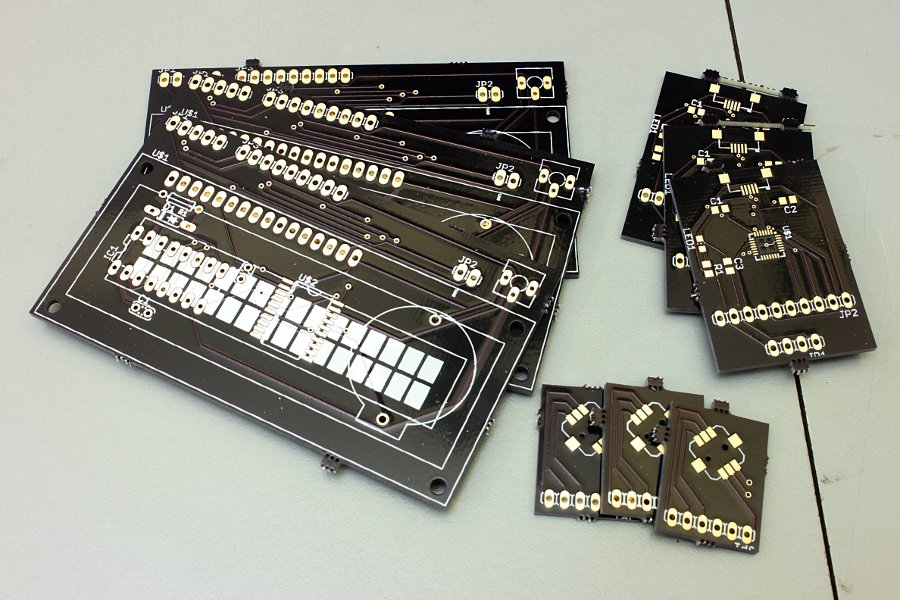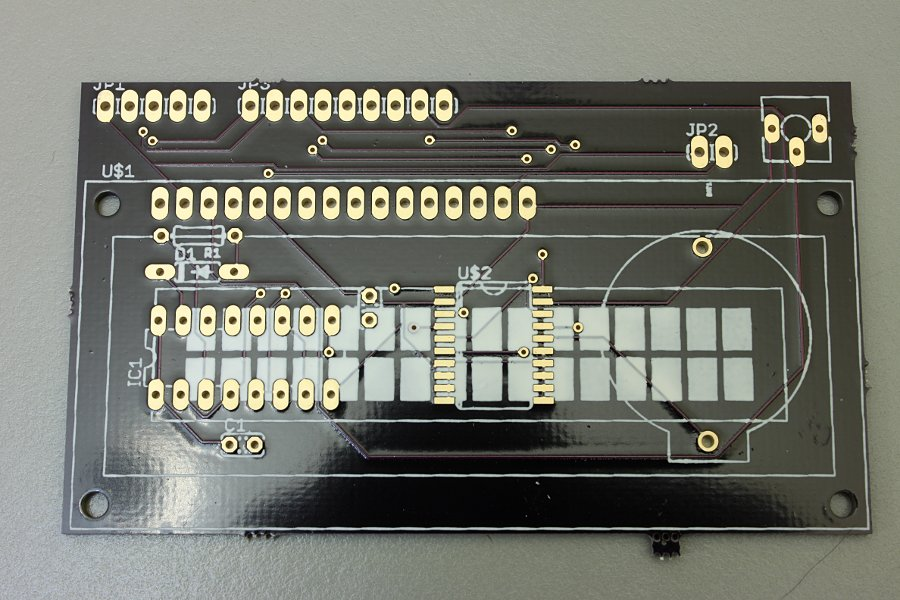Front and back views of the assembled board.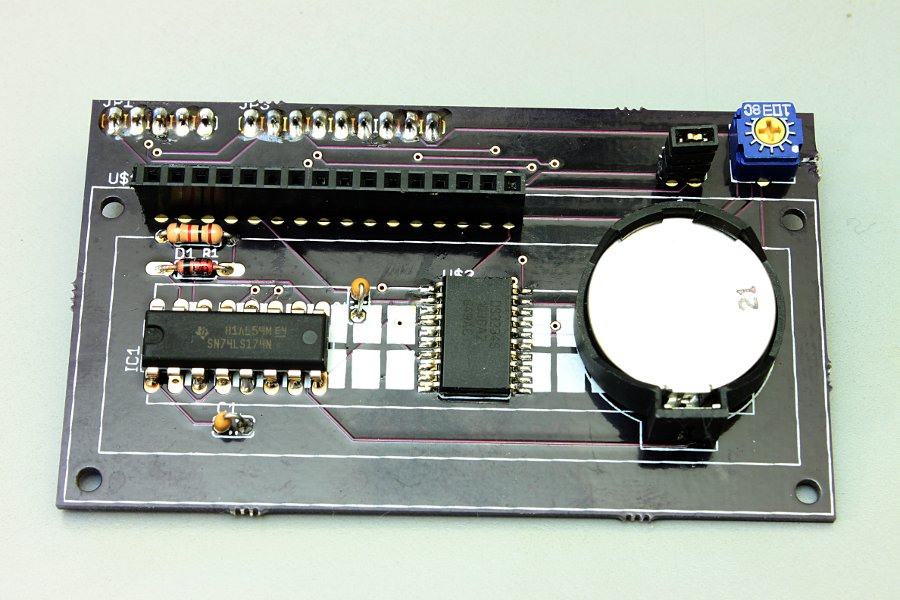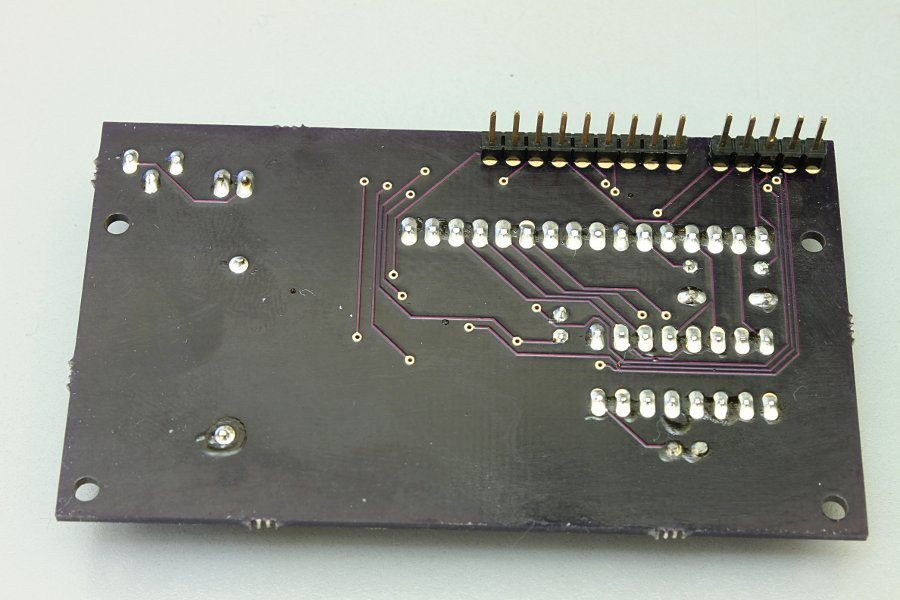Display installed.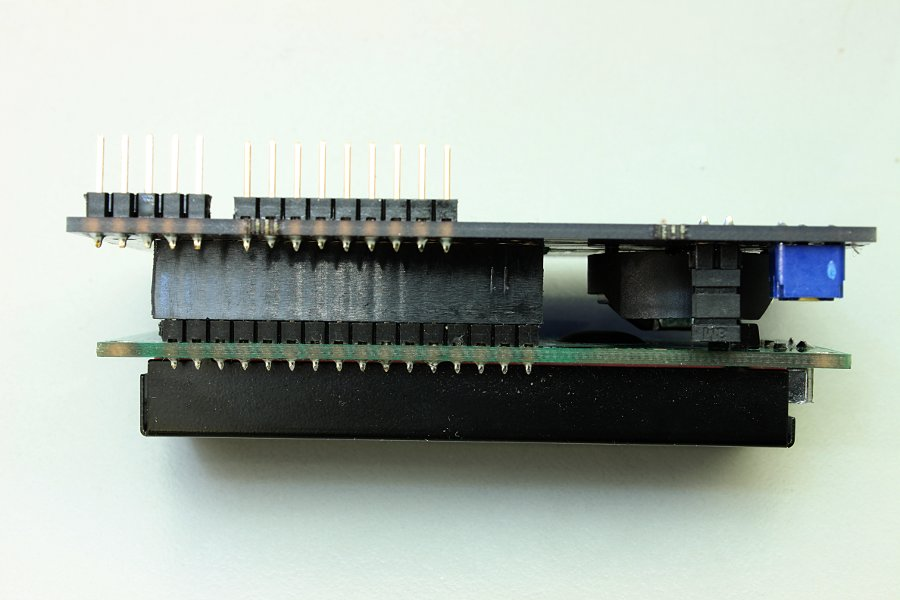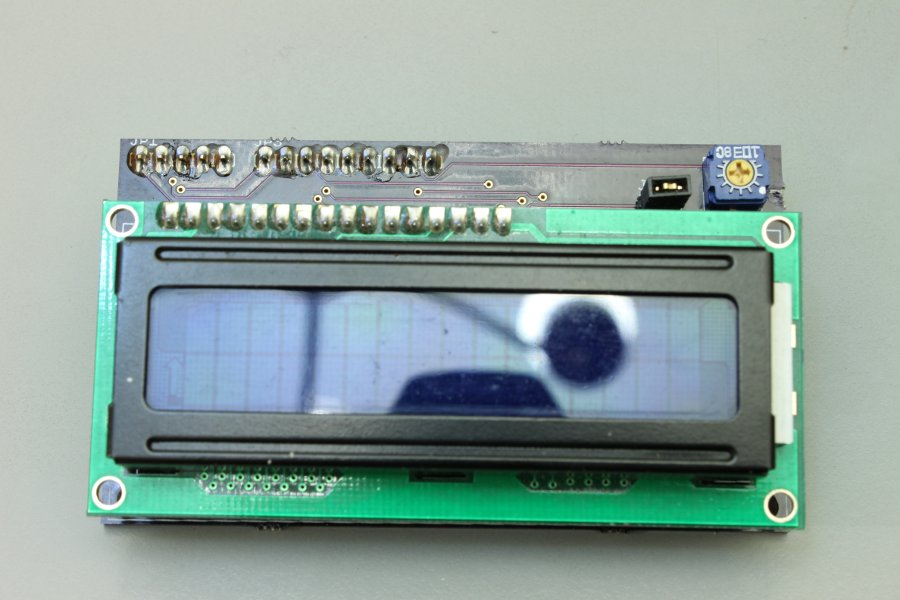Basic testing of the board with an 18F1320.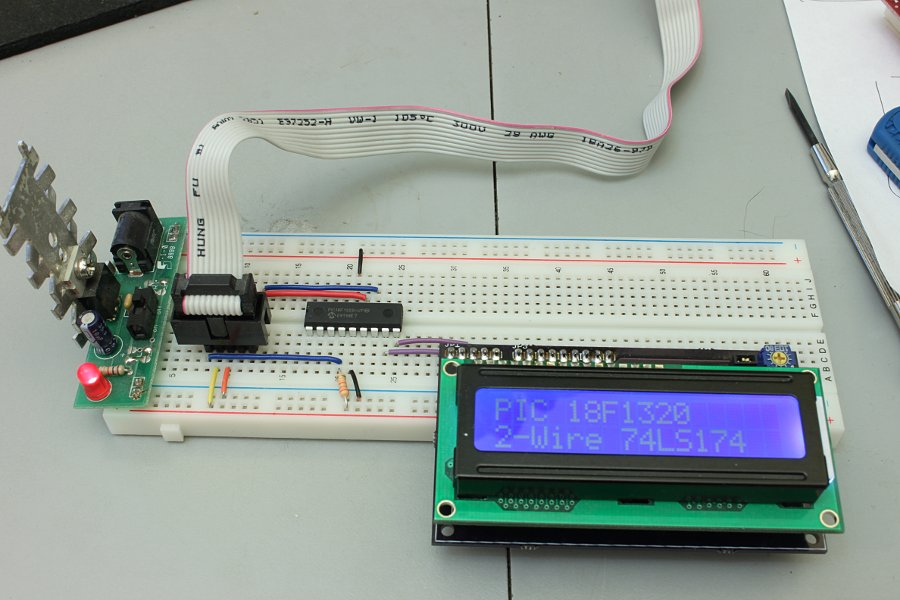Here it's running a clock/thermometer program.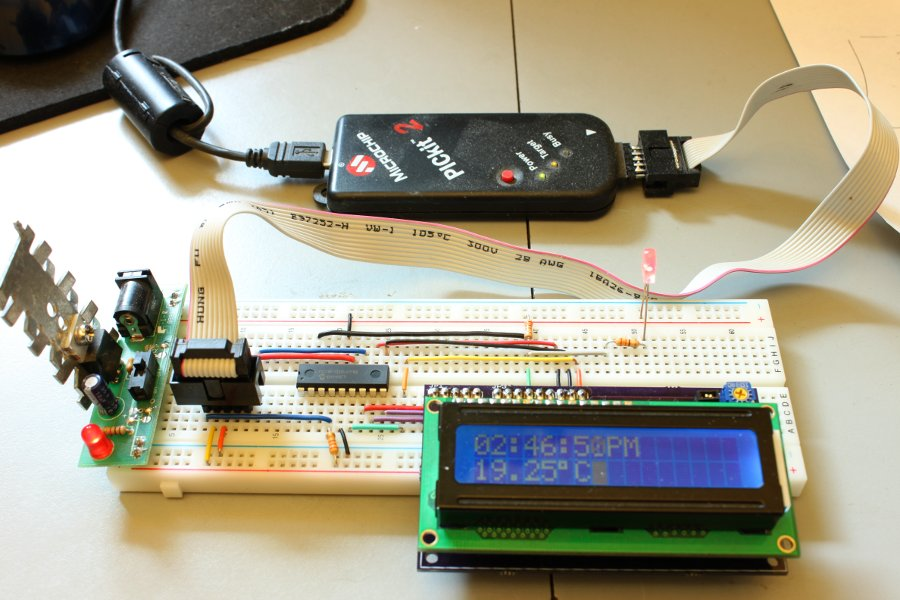I found some proper-size standoffs and screws and installed the display permanently. Much better than hanging off the pins.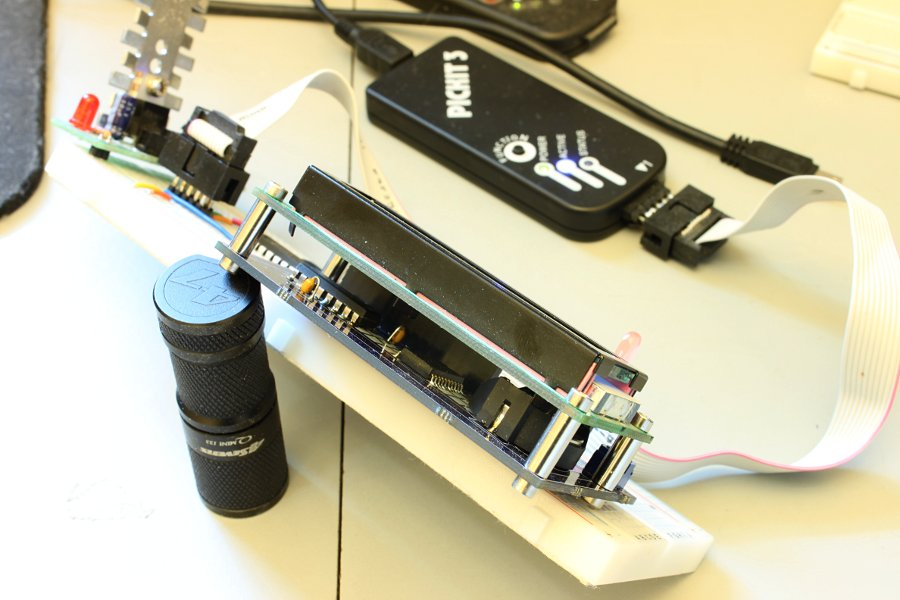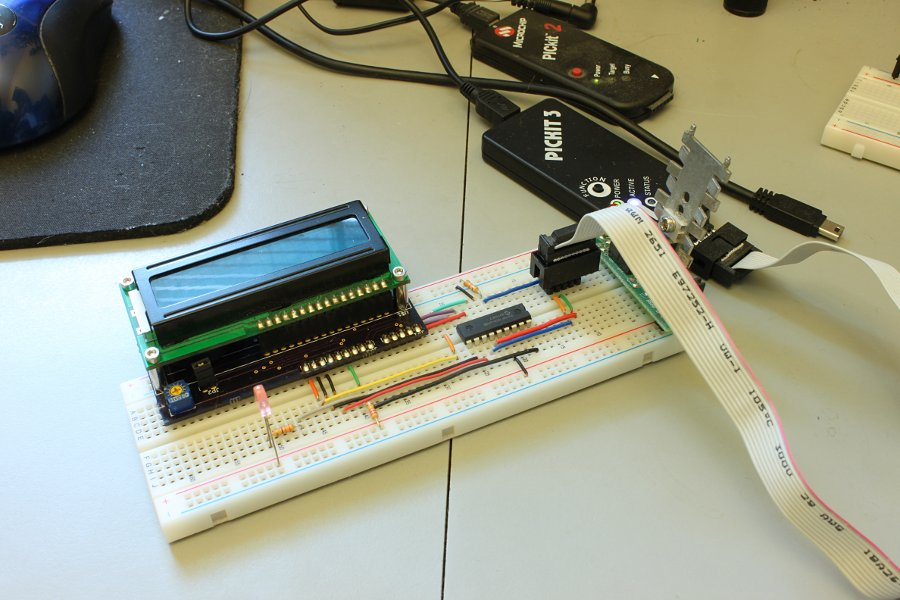If you want to make your own board just download my Eagle schematic and board layout. You'll also need my Eagle library. This file set is for the DorkbotPDX PCB service. You may possibly have to make changes for other PCB services - I don't know.

Here's a pinout diagram for the board (top view):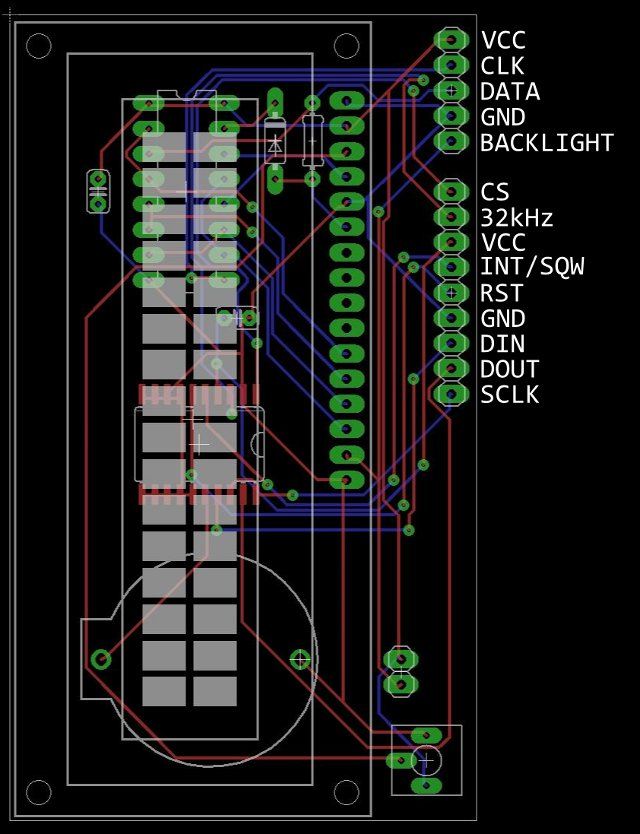Here's the 18F1320 clock/thermometer program that's running in the picture above.

``````#include <system.h>
#include <stdio.h>
#pragma CLOCK_FREQ 8000000
#pragma DATA    _CONFIG1H, _INTIO2_OSC_1H
#pragma DATA    _CONFIG2H, _WDT_OFF_2H
#pragma DATA    _CONFIG3H, _MCLRE_ON_3H
#pragma DATA    _CONFIG4L, _LVP_OFF_4L

//ds3234 functions
void spi_write(unsigned char);
unsigned char busycheck(void);
//display functions
void lcd_cmd(unsigned char);
void lcd_char(char);
void e_togg(void);
void lcd_init(void);
void lcd_nybble(unsigned char,unsigned char);

#define LINE1   lcd_cmd(0x80);
#define LINE2   lcd_cmd(0xc0);
//LCD
#define    CLOCK   latb.0
#define DATA    latb.1
//RTC
#define CLK     lata.2
#define    MOSI    latb.5
#define MISO    portb.4
#define CS      lata.3

char *dayy[]={0,"Sun","Mon","Tue","Wed","Thu","Fri","Sat"};
char string[] = "                ";
unsigned char dflag = 0;

void interrupt(void){
if(intcon3.INT2IF){     //int2 interrupt (RB2
dflag = 1;
intcon3.INT2IF = 0;
}
}

int main(void)
{
unsigned char sec,sec10,min,min10,hour,hour10,temp1,temp2,temp3,temp4;
unsigned char seconds,minutes,hours,temp_h,temp_l,x,y,z,am;
unsigned char day,date,month,year,date_h,date_l,month_h,month_l,year_h,year_l;
osccon = 0b01110010;    //8MHz internal osc
adcon1 = 0x7f;          //all digital
trisa = 0;
trisb = 0b00010100;
CS = 1;
CLOCK = CLK = 0;
intcon3.INT2IE = 1;
intcon.GIE = 1;
lcd_init();
/*    CS = 0;
spi_write(0x80);        //address & write enable
spi_write(0x00);        //set seconds
spi_write(0x49);        //set minutes
spi_write(0x71);        //set 12 hour time & hours
spi_write(0x01);        //set day
spi_write(0x20);        //set date
spi_write(0x03);        //set century/month
spi_write(0x11);        //set year
spi_write(0x00);        //set alarm1 sec
spi_write(0x00);        //set alarm1 min
spi_write(0x00);        //set alarm1 hour
spi_write(0x00);        //set alarm1 day/date
spi_write(0x00);        //set alarm2 min
spi_write(0x00);        //set alarm2 hour
spi_write(0x00);        //set alarm2 day/date
spi_write(0x40);        //enable osc, enable sqw
spi_write(0x00);
CS = 1;*/
while(1){
if(dflag){
CS = 0;                     //read time & date
spi_write(0x00);
CS = 1;

while(busycheck());         //check busy flag till clear
CS = 0;                     //do temperature conversion
spi_write(0x8e);
spi_write(0x60);
CS = 1;
CS = 0;                     //read temp
spi_write(0x11);
CS = 1;

sec = (seconds & 0x0f) + 0x30;          //do time digit prep
sec10 = ((seconds & 0x70) >> 4) + 0x30;
min = (minutes & 0x0f) + 0x30;
min10 = ((minutes & 0x70) >> 4) + 0x30;
hour = (hours & 0x0f) + 0x30;
hour10 = ((hours & 0x10) >> 4) + 0x30;
x = (hours & 0x20) >> 5;
if(x)
am = 0x41;      //A
else
am = 0x50;      //P
//display time
LINE1;
lcd_char(hour10);
lcd_char(hour);
lcd_char(':');
lcd_char(min10);
lcd_char(min);
lcd_char(':');
lcd_char(sec10);
lcd_char(sec);
lcd_char(am);
lcd_char('M');

temp_l >>= 6;                           //do temp digit prep
temp_l *= 25;
temp4 = (temp_l % 10) + 0x30;
temp3 = (temp_l / 10) + 0x30;
temp2 = (temp_h % 10) + 0x30;
temp1 = (temp_h / 10) + 0x30;
//display temp
LINE2;
lcd_char(temp1);
lcd_char(temp2);
lcd_char('.');
lcd_char(temp3);
lcd_char(temp4);
lcd_char(223);
lcd_char('C');

dflag = 0;
}
}
}

unsigned char busycheck(void){
unsigned char x;
CS = 0;
spi_write(0x0f);    //control/status register
CS = 1;
x = (x & 0x04) >> 2;
return x;
}

//*****************
//* spi functions *
//*****************
void spi_write(unsigned char d){
unsigned char temp,x;
for(x=0;x<8;x++){
temp = d << x;
MOSI = temp.7;
CLK = 1; CLK = 0;
}
}

unsigned char x,temp = 0;
for(x=0;x<8;x++){
temp = temp << 1;
CLK = 1;
temp.0 = MISO;
CLK = 0;
}
return(temp);
}

//*****************
//* lcd functions *
//*****************
void lcd_cmd(unsigned char letter)
{
unsigned char temp;
temp = letter;
temp = temp>>4;
lcd_nybble(temp,0);
temp = letter;
temp = temp&0x0f;
lcd_nybble(temp,0);
}

void lcd_char(unsigned char letter)
{
unsigned char temp;
temp = letter;
temp = temp >> 4;
lcd_nybble(temp,1);
temp = letter;
temp = temp & 0x0f;
lcd_nybble(temp,1);
}

void lcd_nybble(unsigned char nyb,unsigned char rs)
{
int i;
DATA = 0;                   //clear the 174
for(i=0;i<6;i++){           //repeat for 6 bits
CLOCK=1;delay_us(50);CLOCK=0;        //write 0's to the 174
}
DATA=1;                     //output the AND value
CLOCK=1;delay_us(50);CLOCK=0;
DATA=rs;                    //output the RS bit value
CLOCK=1;delay_us(50);CLOCK=0;
for(i=0;i<4;i++){           //output the nybble
if((nyb & 0x08) != 0)
DATA=1;
else
DATA=0;
CLOCK=1;delay_us(50);CLOCK=0;
nyb=nyb<<1;
}
e_togg();
}

void lcd_init(void)
{
delay_ms(250);
lcd_nybble(0x03,0);
delay_ms(5);
e_togg();
delay_us(160);
e_togg();
delay_us(160);
lcd_nybble(0x02,0);
delay_us(160);
lcd_cmd(0x28);                    //set 4-bit mode and 2 lines
delay_us(160);
lcd_cmd(0x10);                    //cursor move & shift left
delay_us(160);
lcd_cmd(0x06);                    //entry mode = increment
delay_us(160);
lcd_cmd(0x0d);                    //display on - cursor blink on
delay_us(160);
lcd_cmd(0x01);                    //clear display
delay_ms(30);
}

void e_togg(void)
{
DATA=1;
delay_us(50);
DATA=0;
}``````